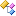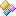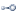﻿ Accord.Math.Distances Namespace# Accord.Math.Distances NamespaceClasses
ClassDescriptionBhattacharyya
Bhattacharyya distance.LogLikelihoodT
Log-likelihood distance between a sample and a statistical distribution.Structures
StructureDescriptionAngular
Angular distance, or the proper distance metric version of Cosine distance.ArgMax
ArgMax distance (L0) distance.BrayCurtis
Bray-Curtis distance.Canberra
Canberra distance.Chebyshev
Chebyshev distance.Cosine
Cosine distance. For a proper distance metric, see Angular.Dice
Dice dissimilarity.DiracT
Dirac distance.Euclidean
Euclidean distance metric.Hamming
Hamming distance.HammingT
Hamming distance.Hellinger
Herlinger distance.Jaccard
Jaccard (Index) distance.JaccardT
Jaccard (Index) distance.Kulczynski
Kulczynski dissimilarity.Levenshtein
Levenshtein distance.LevenshteinT
Levenshtein distance.Mahalanobis
Mahalanobis distance.Manhattan
Manhattan (also known as Taxicab or L1) distance.Matching
Matching dissimilarity.Minkowski
The Minkowski distance is a metric in a normed vector space which can be considered as a generalization of both the Euclidean distance and the Manhattan distance.Modular
Modular distance (shortest distance between two marks on a circle).PearsonCorrelation
Pearson Correlation similarity.RogersTanimoto
Rogers-Tanimoto dissimilarity.RusselRao
Russel-Rao dissimilarity.SokalMichener
Sokal-Michener dissimilarity.SokalSneath
Sokal-Sneath dissimilarity.SquareEuclidean
Square-Euclidean distance and similarity. Please note that this distance is not a metric as it doesn't obey the triangle inequality.SquareMahalanobis
Squared Mahalanobis distance.WeightedEuclidean
Weighted Euclidean distance metric.WeightedSquareEuclidean
Weighted Square-Euclidean distance and similarity. Please note that this distance is not a metric as it doesn't obey the triangle inequality.Yule
Yule dissimilarity.Interfaces
InterfaceDescriptionIDistance
Common interface for distance functions (not necessarily metrics).IDistanceT
Common interface for distance functions (not necessarily metrics).IDistanceT, U
Common interface for distance functions (not necessarily metrics).IMetricT
Common interface for Metric distance functions.ISimilarityT
Common interface for similarity measures.ISimilarityT, U
Common interface for similarity measures.